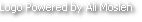# Neural Network - HRK-BRK

HRK-BRK
Go to content

## Neural Network

We Think Different

Computers and brains "think" in completely different ways.
In a computer  the logic gates are wired together and not more than some couples touches each other. In a brain the neurons are connected in parallel ways, each single cells is wired with thousand of neighbors. The main difference it is how they work: the first are synchronized basically (there are exception from the macroscopic point) in serial , the second works in parallel. It could fits as comparison: the same difference we can find in programming languages, for example Java/C++ a vs VHDL.
They share the numbers of components but not the mechanism to manage the information

Neural neworks are organized in interconnected inside different layers.

The nodes are not passive but "live" and wait the moment to became active (activation function).
Related to each task they have some "weights".

As an example we can image a simple network composed by three layers to figure out an intelligent device that looks (input) some information (colors), elaborate (hidden layers) and react (output layer):

a) The input layer, this plays the role of sensors

b) The hidden layer, this is the "state" or memory

c) The output layer, this is the actuator (the action)

As an example we can image a simple network composed by three layers to figure out an intelligent device that respond to (input) some information (colors), elaborate (hidden layers) and react (output layer):

a) The input layer, this  plays the role of sensors

b) The hidden layer, this is the "state" or memory

c) The output layer, this is the actuator (the action)

In the middle of this process there is the 'learning' phase. NNs work across a learning process that correct at each cycle the output.

Every epoch, when new inputs trigger a new task the weights modify and correct their errors until the output state remain stable

The learning process "copies" what happens in the biological system

Neural networks have been applied to a wide variety of different areas:

• speech synthesis
• pattern recognition
• diagnostic problems
• medical illnesses
• robotic control
• computer visionBack to content